# Absolute Value of a Number

## Introduction

In this arti­cle we are going to see the absolute val­ue func­tion of a num­ber, what are its prop­er­ties, some graph­ics and as an extra we are going to see how to cal­cu­late the absolute val­ue of an expres­sion in the Uni­ty graph­ic engine, in the field of pro­gram­ming and game development.

# MOST SEARCHED VIDEOS FROM MY CHANNEL

## Definition of the Absolute Value of a Number

The absolute val­ue of a num­ber is the dis­tance between that num­ber and the 0 of the mea­sure­ment system.

This results in two pos­si­ble out­comes depend­ing on the num­ber in question.

If the num­ber is pos­i­tive, the result of tak­ing the absolute val­ue is the same num­ber. If on the oth­er hand the num­ber is neg­a­tive, when tak­ing the absolute val­ue the result is the same num­ber but in pos­i­tive value.

For­mal­ly: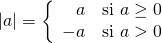Because of this def­i­n­i­tion we can affirm that the absolute val­ue of a num­ber is always a pos­i­tive number.

## Graph of the Absolute Value of X

Below are some graphs of the absolute val­ue func­tion to under­stand how it behaves.

Observe in each graph the ranges of the vari­ables in the axes to see how the graph is affect­ed by the dis­place­ments in X in Y and by the coef­fi­cients that mul­ti­ply the expression.

(1)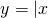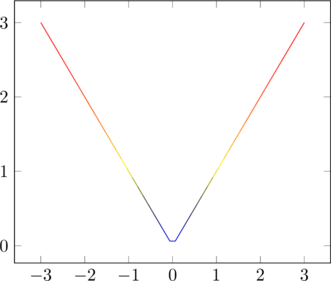(2)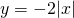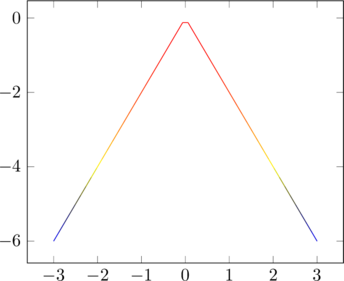(3)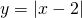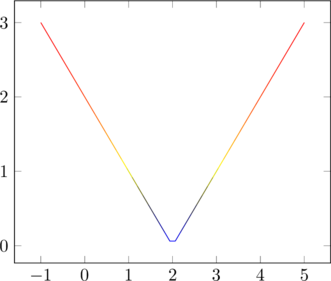(4)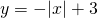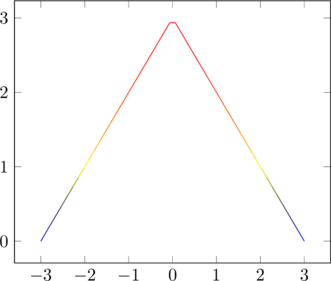## Distances

When we are work­ing with dif­fer­ent points in a coor­di­nate sys­tem, we are often inter­est­ed in work­ing with the dis­tances between them, which are pos­i­tive values.

The absolute val­ue allows work­ing with these dis­tances in math­e­mat­i­cal expressions.

## How to calculate the Absolute Value in Unity

To cal­cu­late the absolute val­ue of an expres­sion in Uni­ty, we must make use of the sta­t­ic method "Abs" of the class "Mathf".

Let "num" be a numer­i­cal vari­able defined in the pro­gram in ques­tion, to take its absolute val­ue we do:

Mathf.Abs(num);

The result of the exe­cu­tion of this method can be stored in anoth­er vari­able or in the same one, in the fol­low­ing way:

num = Mathf.Abs(num);

Scroll to Top
Secured By miniOrange